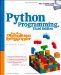# Using Python Classes in C

In the previous chapter, we saw how to use C++ classes in Python by wrapping them with SWIG. But what about going the other way -- using Python classes from other languages? It turns out that this is really just a matter of applying interfaces already shown.

Recall that Python scripts generate class instance objects by calling class objects as though they were functions. To do it from C (or C++), you simply follow the same steps: import a class from a module (or elsewhere), build an arguments tuple, and call it to generate an instance using the same C API tools you use to call Python functions. Once youve got an instance, you can fetch attributes and methods with the same tools you use to fetch globals out of a module.

To illustrate how this works in practice, Example 20-12 defines a simple Python class in a module that we can utilize from C.

##### Example 20-12. PP2EIntegrateEmbedApiClientsmodule.py
```# call this class from C to make objects

class klass:
def method(self, x, y):
return "brave %s %s" % (x, y) # run me from C```

This is nearly as simple as it gets, but its enough to illustrate the basics. As usual, make sure that this module is on your Python search path (e.g., in the current directory, or one listed on your PYTHONPATH setting), or else the import call to access it from C will fail, just as it would in a Python script. Now, here is how you might make use of this Python class from a Python program:

```C:...PP2EIntegrateEmbedApiClients>python
>>> import module # import the file
>>> object = module.klass( ) # make class instance
>>> result = object.method(sir,
obin) # call class method
>>> print result
brave sir robin```

This is fairly easy stuff in Python. You can do all these operations in C too, but it takes a bit more code. The C file in Example 20-13 implements these steps by arranging calls to the appropriate Python API tools.

##### Example 20-13. PP2EIntegrateEmbedApiClientsobjects-low.c
```#include
#include

main( ) {
/* run objects with low-level calls */
char *arg1="sir", *arg2="robin", *cstr;
PyObject *pmod, *pclass, *pargs, *pinst, *pmeth, *pres;

/* instance = module.klass( ) */
Py_Initialize( );
pmod = PyImport_ImportModule("module"); /* fetch module */
pclass = PyObject_GetAttrString(pmod, "klass"); /* fetch module.class */
Py_DECREF(pmod);

pargs = Py_BuildValue("( )");
pinst = PyEval_CallObject(pclass, pargs); /* call class( ) */
Py_DECREF(pclass);
Py_DECREF(pargs);

/* result = instance.method(x,y) */
pmeth = PyObject_GetAttrString(pinst, "method"); /* fetch bound method */
Py_DECREF(pinst);
pargs = Py_BuildValue("(ss)", arg1, arg2); /* convert to Python */
pres = PyEval_CallObject(pmeth, pargs); /* call method(x,y) */
Py_DECREF(pmeth);
Py_DECREF(pargs);

PyArg_Parse(pres, "s", &cstr); /* convert to C */
printf("%s
", cstr);
Py_DECREF(pres);
}```

Step through this source file for more details; its merely a matter of figuring out how you would accomplish the task in Python, and then calling equivalent C functions in the Python API. To build this source into a C executable program, run the makefile in the files directory (its analogous to makefiles weve already seen). After compiling, run it as you would any other C program:

```[mark@toy ~/.../PP2E/Integrate/Embed/ApiClients]\$ objects-low
brave sir robin```

This output might seem anticlimactic, but it actually reflects the return values sent back to C by the class method in file module.py. C did a lot of work to get this little string: it imported the module, fetched the class, made an instance, and fetched and called the instance method, performing data conversions and reference count management every step of the way. In return for all the work, C gets to use the techniques shown in this file to reuse any Python class.

Of course, this example would be more complex in practice. As mentioned earlier, you generally need to check the return value of every Python API call to make sure it didn fail. The module import call in this C code, for instance, can fail easily if the module isn on the search path; if you don trap the NULL pointer result, your program will almost certainly crash when it tries to use the pointer (at least eventually). Example 20-14 is a recoding of Example 20-13 with full error-checking; its big, but its robust.

##### Example 20-14. PP2EIntegrateEmbedApiClientsobjects-err-low.c
```#include
#include
#define error(msg) do { printf("%s
", msg); exit(1); } while (1)

main( ) {
/* run objects with low-level calls and full error checking */
char *arg1="sir", *arg2="robin", *cstr;
PyObject *pmod, *pclass, *pargs, *pinst, *pmeth, *pres;

/* instance = module.klass( ) */
Py_Initialize( );
pmod = PyImport_ImportModule("module"); /* fetch module */
if (pmod == NULL)

pclass = PyObject_GetAttrString(pmod, "klass"); /* fetch module.class */
Py_DECREF(pmod);
if (pclass == NULL)
error("Can	 get module.klass");

pargs = Py_BuildValue("( )");
if (pargs == NULL) {
Py_DECREF(pclass);
error("Can	 build arguments list");
}
pinst = PyEval_CallObject(pclass, pargs); /* call class( ) */
Py_DECREF(pclass);
Py_DECREF(pargs);
if (pinst == NULL)
error("Error calling module.klass( )");

/* result = instance.method(x,y) */
pmeth = PyObject_GetAttrString(pinst, "method"); /* fetch bound method */
Py_DECREF(pinst);
if (pmeth == NULL)
error("Can	 fetch klass.method");

pargs = Py_BuildValue("(ss)", arg1, arg2); /* convert to Python */
if (pargs == NULL) {
Py_DECREF(pmeth);
error("Can	 build arguments list");
}
pres = PyEval_CallObject(pmeth, pargs); /* call method(x,y) */
Py_DECREF(pmeth);
Py_DECREF(pargs);
if (pres == NULL)
error("Error calling klass.method");

if (!PyArg_Parse(pres, "s", &cstr)) /* convert to C */
error("Can	 convert klass.method result");
printf("%s
", cstr);
Py_DECREF(pres);
}

```Python Programming for the Absolute Beginner, 3rd Edition
ISBN: 1435455002
EAN: 2147483647
Year: 2000
Pages: 245